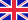•# Voltage

Autor: Lambert M. Surhone

Voltage is commonly used as a short name for electrical potential difference. Its corresponding SI unit is the volt (symbol: V, not italicized). Electric potential is a hypothetically measurable physical dimension, and is denoted by the algebraic variable... Viac o knihe

Na objednávku, dodanie 2-4 týždne

29.75 €

bežná cena: 35.00 €

## O knihe

Voltage is commonly used as a short name for electrical potential difference. Its corresponding SI unit is the volt (symbol: V, not italicized). Electric potential is a hypothetically measurable physical dimension, and is denoted by the algebraic variable V (italicized). The voltage between two (electron) positions 'A' and 'B', inside a solid electrical conductor (or inside two electrically-connected, solid electrical conductors), is denoted by (VA - VB). This voltage is the electrical driving force that drives a conventional electric current in the direction A to B. Voltage can be directly measured by an 'ideal voltmeter'. Well-constructed, correctly used, real voltmeters approximate very well to ideal voltmeters. For non-scientists, an analogy involving the flow of water is sometimes helpful in understanding the concept of voltage (see below).

• Vydavateľstvo: Betascript Publishers
• Rok vydania: 2009
• Formát: Paperback
• Rozmer: 220 x 150 mm
• Jazyk: Anglický jazyk
• ISBN: 9786130309299

Generuje redakčný systém BUXUS CMS spoločnosti ui42.